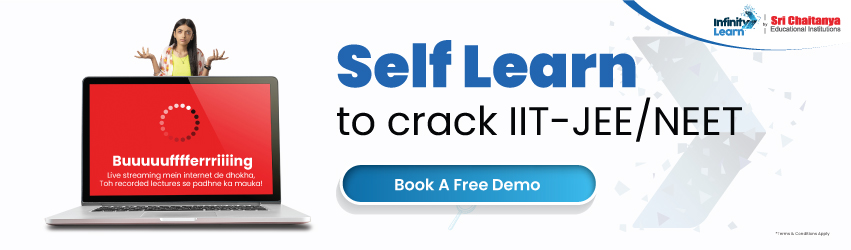NCERT Solutions for Class 12 Maths Chapter 7 Integrals (Ex 7.4) Exercise 7.4

# NCERT Solutions for Class 12 Maths Chapter 7 Integrals (Ex 7.4) Exercise 7.4infinitylearn surge self learn
infinitylearn starter package

Integration is one of the most significant topics in the 12th-grade math curriculum. As a result, Infinity Learn’s highly experienced experts created the Exercise 7.4 Class 12 Maths NCERT Solution PDF to assist students in understanding the thorough approach of solving integration sums. Infinity Learn offers free downloads of Class 12 Maths Chapter 7 Exercise 7.4 Solutions PDF. These solutions will assist students in grasping all of the chapter’s important themes. As a result, pupils will be able to achieve the maximum possible grades on their tests.

Do you need help with your Homework? Are you preparing for Exams? Study without Internet (Offline)
×
Exercise 7.4

Need FREE NCERT/CBSE/IIT-JEE/NEET Study Material?

Class 12 Maths NCERT Solutions For Chapter 7 Exercise 7.4

Integral is a challenging chapter, and students will gain a better knowledge of the sorts of sums discussed in integration by studying the Ex 7.4 Class 12 NCERT Solutions PDF on Infinity Learn. However, there are key elements that students should be familiar with before going through Class 12 Maths NCERT Solutions Chapter 7 Exercise 7.4. We’ll go through those crucial principles in this part.

Ex 7.4 Solutions for Class 12 Maths include solutions to all of the sums in this exercise. Integration is defined in this chapter as a method for determining the areas of two-dimensional regions and computing the volumes of three-dimensional objects.

As a result, if someone wants to find the integral of a function with respect to x, they must first calculate the area to the x-axis from the curve. Integrals are also known as antiderivatives by some experts. This is due to the fact that integration is the inverse of differentiation.

Integrals come in a variety of shapes and sizes. In Maths NCERT Solutions Class 12 Chapter 7 Exercise 7.4, students will learn about the two types of integrals.

The following are the two types of integrals.

• Definite Integral
• Indefinite Integral

Integrals can also be used in a variety of ways. Integrals are used in the following ways in mathematics:

• To determine a curve’s average value.
• To find the area beneath a curve.
• To calculate the area of any two curves.
• To calculate the centroid’s center of mass if the region has curved sides.

Download Ex 7.4 Class 12 Maths NCERT Solutions PDF from Infinity Learn

In mathematics, the integral is a crucial chapter. Infinity Learn has solved NCERT Solutions PDF that you may download and refer to.

Apart from exercise 7.4, the maths NCERT book contains other exercises such as 7.1, 7.2, 7.3, 7.5, 7.6, 7.7, 7.8, 7.9, 7.10, and exercise 7.11. All of the questions in the previous exercises should be answered by the students. We at Infinity Learn provide solutions to all of the chapter 7 class 12th exercises. These solutions’ PDF files are free to download.

Class 12 Maths Chapter 7 Exercise 7.4 NCERT Solutions Benefits

The following are some of the advantages of using NCERT solutions for Exercise 7.4

in-class 12 maths:

• All of the tasks in the book have Infinity Learn NCERT maths solutions, which are helpful in relieving pressure on students.
• All students can download and print these solutions to make them more convenient.
• The solutions were written by professional math teachers to ensure accuracy.
• Infinity Learn provides solutions that are completely free of charge.

FAQ:

1. Where can I find solved NCERT Solutions for Chapter 7 of Class 12 Maths?

Ans. Students may access solved NCERT Solutions for Class 12 Maths on Infinity Learn’s official website. It’s an online learning platform that gives pupils a variety of solutions and notes to help them comprehend things better. Our subject-matter specialists, who have years of experience in this industry, created these solutions.

1. What are the themes covered in Exercise 7.4 of Chapter 7 of Class 12 Maths?

Ans. Integrals are the title of Chapter 7 in Class 12 Math. While the rest of the chapter covers numerous methods for solving integration sums, Exercise 7.4 focuses on the two types of integrals and the method for computing the areas of two-dimensional regions and finding the volumes of three-dimensional objects. Students should be able to solve the sums in Exercise 7.4 using the procedures taught in class.

1. Should I practice all of the examples from Chapter 7 of Class 12 Maths, Exercise 7.4?

Ans. The syllabus for Class 12 Maths includes a lot of examples. All of the examples are based on the exercises that follow, and practicing them will aid you in solving questions in the exercise. All students should practice all of the examples in Exercise 7.4 of Chapter 7 of Class 12 Maths since they will help them understand the methods and ideas better. Exam questions frequently include questions based on examples.

Join Infinity Learn Regular Class Programme!